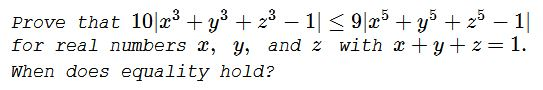# Problem 11804 from the AMM

### Problem### Solution 1

Since $x+y+z=1,\;$ we have the following identities:

\displaystyle\begin{align} x^3+y^3+z^3-1 &= x^3+y^3+z^3+(x+y+z)^3\\ &=-3(x+y)(y+z)(z+x),\\ x^5+y^5+z^5-1 &= x^5+y^5+z^5-(x+y+z)^5\\ &=-\frac{5}{2}(x+y)(y+z)(z+x)\left[(x+y)^2+(y+z)^2+(z+x)^2\right]. \end{align}

Thus, if the denominator is nonzero, we obtain

\displaystyle\begin{align} \frac{|x^5+y^5+z^5-1|}{|x^3+y^3+z^3-1|} &= \frac{5}{6}\cdot\left[(x+y)^2+(y+z)^2+(z+x)^2\right]\\ &\ge \frac{5}{6}\frac{[2(x+y+z)]^2}{3}=\frac{10}{9}. \end{align}

Equality holds if and only if $\displaystyle x=y=z=\frac{1}{3},\;$ or $(x,y,z)\;$ is a permutation of $(t,-t,1)\;$ for some $t\in\mathbb{R}.$

### Solution 2

Due to the constraint $x+y+z=1,\;$ the required inequality is a consequence of the relation

\displaystyle\begin{align} 10^2(x^3+y^3+z^3-1)^2 &\le 10^2(x^3+y^3+z^3-(x+y+z)^3)^2(x+y+z)^4\\ &+\frac{225}{4}(x+y)^2(y+z)^2(z+x)^2\\ &\;\;\;\cdot ((x-y)^2+(y-z)^2+(z-x)^2)\\ &\;\;\;\cdot (3(x^2+y^2+z^2)+7(x+y+z)^2)\\ &=9^2(x^5+y^5+z^5-(x+y+z)^5)^2. \end{align}

Equality holds for the cases listed in the first solution.

### Solution 3

Introduce $a=xy+yz+zx\;$ and $b=xyz.\;$ This makes $x,y,z\;$ the roots of the polynomial $f(t)=t^3-t^2+at-b.\;$ By the Vi&e\grave;te-Newton formulas,

$\displaystyle\sum_{cycl}x^3=3(b-a)+1\\ \displaystyle\sum_{cycl}x^5=5(b-a)(1-a)+1.$

Thus the task becomes to prove $30|b-a|\le 45|(b-a)(1-a)|,\;$ i.e., $4(b-a)^2\le 9[(b-a)(1-a)]^2,\;$ which reduces to

$(b-a)^2(1-3a)(5-3a)\ge 0.$

Obviously $(b-a)^2\ge 0.\;$ Now, since $f(t)\;$ has three real roots, then by Rolle's theorem, $f'(t),\;$ which is a quadratic polynomial, has two real roots, meaning that its discriminant is not negative: $1-3a\ge 0.\;$ This, in turn, implies $5-3a\gt 0,$ thus proving the required inequality.

Let's turn to the cases of equality. If $1-3a=0,\;$ then $\displaystyle f(t)=\left(t-\frac{1}{3}\right)^3-\left(b-\frac{1}{27}\right),\;$ implying $\displaystyle b=\frac{1}{27},\;$ and, consequently, $\displaystyle x=y=z=\frac{1}{3}.\;$ If $b=a,\;$ then $f(t)=(t-1)(t^2+a).\;$ Hence, $a\le 0\;$ and $\{x,y,z\}=\{1,m,-m\},\;$ where $m\in\mathbb{R}.$

### Solution 4

We replace $z\;$ with $1-x-y.\;$ First we remark that

\begin{align} LHS &= 10|x^3+y^3+(1-x-y)^3-1|\\ &=30|x-1||y-1||x+y| \end{align}

and

\begin{align} RHS &= 9|x^5+y^5+(1-x-y)^5-1|\\ &=45|x-1||y-1||x+y|\cdot\frac{1}{2}(2+(x+y)^2+(x-1)^2+(y-1)^2). \end{align}

Thus the inequality can be writen as

$\displaystyle |x-1||y-1||x+y|\left[\left(x-\frac{1}{3}\right)^2+\left(y-\frac{1}{3}\right)^2+\left(\left(x-\frac{1}{3}\right)+\left(y-\frac{1}{3}\right)\right)^2\right]\ge 0.$

From this we see that the required inequality holds for all $(x,y)\;$ and that we have equality for $(x,y,z)=(1,t,-t),\;$ $(x,y,z)=(t,1,-t),\;$ $(x,y,z)=(t,-t,1),\;$ and $\displaystyle (x,y,z)=\left(\frac{1}{3},\frac{1}{3},\frac{1}{3}\right),\;$

### Acknowledgment

The first two solutions have been lifted from the American Mathematical Monthly. The first solution is by George Stoica (Canada); the second is by Oliver Geupel (Germany); Solution 3 is by Leo Giugiuc (Romania), who brought this problem to my attention, in the first place, Solution 4 is by Hansruedi Widmer (Switzerland).

Reference

1. Problems and Solutions, Am Math Monthly, 123, October 2016, pp 832-833